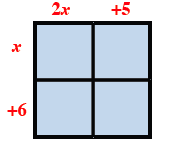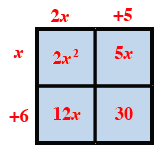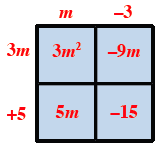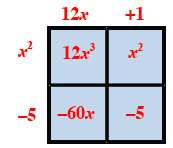Home > CAAC > Chapter 5 > Lesson 5.1.3 > Problem5-27

5-27.

Use a generic rectangle to multiply the following expressions. Write each solution both as a sum and as a product. Homework Help ✎

1. $(2x+5)(x+6)$

• Draw a generic rectangle and label the length and width.

$(2x+5)(x+6)=2x^2+17x+30$Fill in the grid by multiplying the length and width of each box.

Write an equation showing the product is the sum of the parts.
Area as a sum: $2x^2+12x+5x+30=2x^2+17x+30$
Area as a product: $(2x+5)(x+6)$

1. $(m−3)(3m+5)$

• See the help for part (a).Area as product: $(m−3)(3m+5)$
Area as sum: $3m^2−4m−15$

1. $(12x+1)(x^2−5)$

• See the help for part (a).1. $(3−5y)(2+y)$

• See the help for part (a).